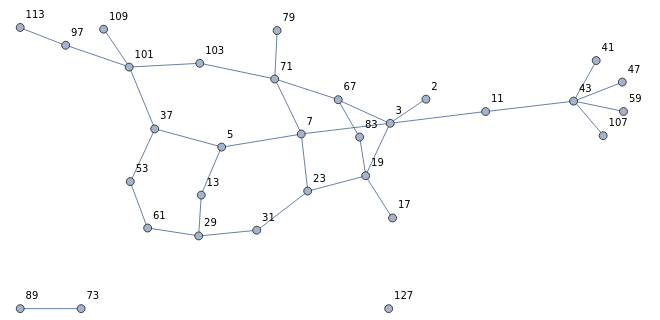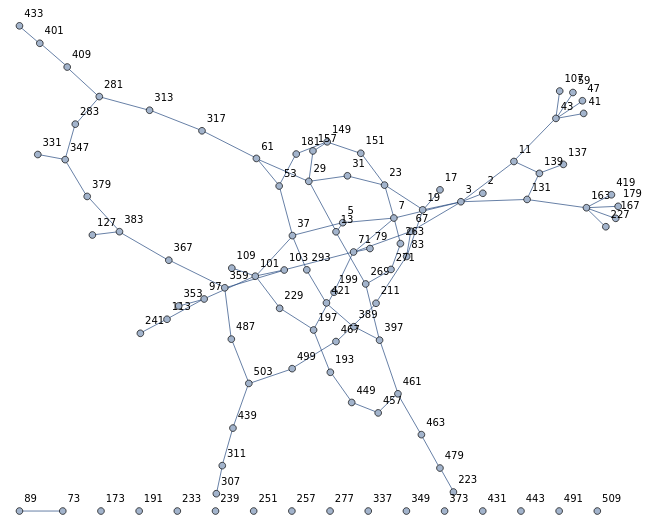# Hamming cube of primes

Given two nonnegative integers, m and n, we say that they are Hamming-adjacent if and only if their binary expansions differ in exactly one digit. For example, the numbers 42 and 58 are Hamming-adjacent because their binary expansions 101010 and 111010 differ in a single position.

If m and n are Hamming-adjacent, then their absolute difference |n − m| is necessarily a power of 2. The converse is not true, though; 24 and 32 have a difference of 8, but their binary expansions 011000 and 100000 differ in three positions.

We can form a countably infinite graph G on the set of all nonnegative integers by connecting two vertices if and only if they’re Hamming-adjacent. G is a bipartite graph: two integers can only be Hamming-adjacent if one is odious and the other is evil.

G is the union of nested hypercube graphs: the first$2^d$ nonnegative integers form a hypercube graph of degree d. For example, here’s the subgraph induced by the first 32 naturals:What about if we take the induced subgraph on only the vertices which are primes? For the primes below 32, we get the following graph:It’s a connected graph! It remains connected if we repeat for the primes below 64:What about for the primes below 128? Now it has an isolated vertex, 127, and an isolated edge:When we go further and look at the primes below 512, the vertex 127 is no longer isolated; it has been connected into the main component via the prime 383:Similarly, the edge between 89 and 73 becomes assimilated into the main component once we increase our horizon to the primes below 1024.

This raises the question: does every prime eventually become connected to the main component? Or, equivalently, when we form the countably infinite induced subgraph H of G whose vertices are all of the primes, is H connected?

### Isolated vertices

It turns out that the answer is no: the prime 2131099 is an isolated vertex in H with no neighbours whatsoever! (It is plausibly the smallest such example.)

How does one even prove that 2131099 is an isolated vertex? In other words, how do we show that all of the Hamming-adjacent integers are composite? Firstly, note that the Hamming-adjacent integers smaller than itself are a finite set obtained by subtracting one of the powers of 2 in its binary expansion:

33947, 2098331, 2130075, 2130971, 2131083, 2131091, 2131097, 2131098

These can all be verified to be composite. But what about the infinitely many Hamming-adjacent integers that are larger than itself? These are necessarily of the form $2131099 + 2^k$ for some value k. It transpires that every element in this set must be divisible by at least one of the primes {3, 5, 7, 13, 17, 241}, called the covering set. In particular, we have:

• k = 1 (mod 2) implies divisible by 3;
• k = 0 (mod 4) implies divisible by 5;
• k = 1 (mod 3) implies divisible by 7;
• k = 2 (mod 12) implies divisible by 13;
• k = 2 (mod 8) implies divisible by 17;
• k = 6 (mod 24) implies divisible by 241;

and together these cover all residue classes modulo 24.

We can go further and show that there are infinitely many such isolated vertices. In particular, every number of the following form:

n = 1885107369798300628981297352055 h + 3316923598096294713661

has a covering set of primes for all numbers of the form$n \pm 2^k$, as discovered by Christophe Clavier. Specifically, there are two covering sets of primes: one for the ‘+’ case and one for the ‘−’ case; the union of these two sets is just the set:

{3,5,7,11,13,17,19,31,37,41,73,97,109,151,241,331,673,1321}

of prime divisors of the linear coefficient.

So, we just need to show that there are infinitely many primes of this form that are not Hamming-adjacent to any of the primes in the above set. The latter condition is easy to enforce — for example, by insisting that n is congruent to 4095 mod 4096 (i.e. its binary expansion ends in twelve ‘1’s). Then we are left with an arithmetic progression whose offset and common difference are coprime, and a theorem of Dirichlet states that there are infinitely many primes in such an arithmetic progression.

### A difficult conjecture

Given the existence of infinitely many isolated vertices, we postulate the following conjecture:

Conjecture: Other than the infinitely many isolated vertices, there is exactly one connected component in H.

What would a counterexample look like? The smallest such counterexample would be a pair of Hamming-adjacent primes with p > q and no further neighbours. The usual method of covering sets would only work under the following set of conditions:

1. p has a covering set P preventing any larger Hamming-adjacent primes;
2. q has a covering set Q preventing any larger Hamming-adjacent primes, with the single exception of p, and this is only possible if p is itself a member of Q;
3. The finitely many numbers smaller than and Hamming-adjacent to p all happen to be composite, with the single exception of q;
4. The finitely many numbers smaller than and Hamming-adjacent to q all happen to be composite.

The second of these conditions is incredibly implausible, because the primes appearing in a covering set are usually much smaller than the dual Sierpinski numbers that arise from such a covering set. Here we are asking for the prime in the covering set to be larger!

Note that this conjecture is definitely unsolved, because it’s stronger than the conjecture that there are primes of every Hamming weight.

This entry was posted in Uncategorized. Bookmark the permalink.

### 2 Responses to Hamming cube of primes

1.Simon Tatham says:

I’m confused by the final paragraph. If conjecture A is stronger than conjecture B, and B is known to be unsolved, that certainly implies that A is unproved, but surely not that it’s unsolved. The stronger conjecture might have been disproved, without producing a solution one way or the other to the weaker one, might it not?

•apgoucher says:

Yes, you’re correct: if P and Q are conjectures, and Q is stronger than P, then it’s entirely possible that Q has been resolved and P has not (because Q has been proved false!).